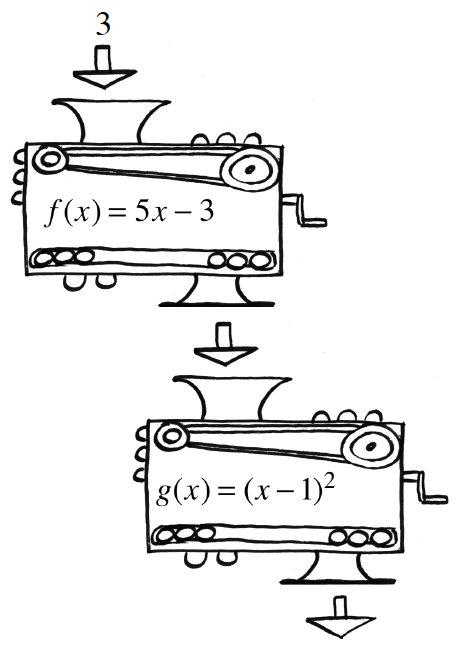### Home > INT3 > Chapter 5 > Lesson 5.1.3 > Problem5-46

5-46.Two function machines, $f(x) = 5x - 3$ and $g(x) = (x - 1)^2$, are shown at right.

1. Suppose $f(3)$, not $x = 3$, is dropped into the $g(x)$ machine. This is written as $g(f(3))$. What is this output?

$f(3) = 12$
so $g(f(3)) = g(12) = 121$

2. Using the same function machines, what is $f(g(3))$? Be careful! The result is different from the last one because the order in which you use the machines has been switched!

$f(g(3)) = 17$